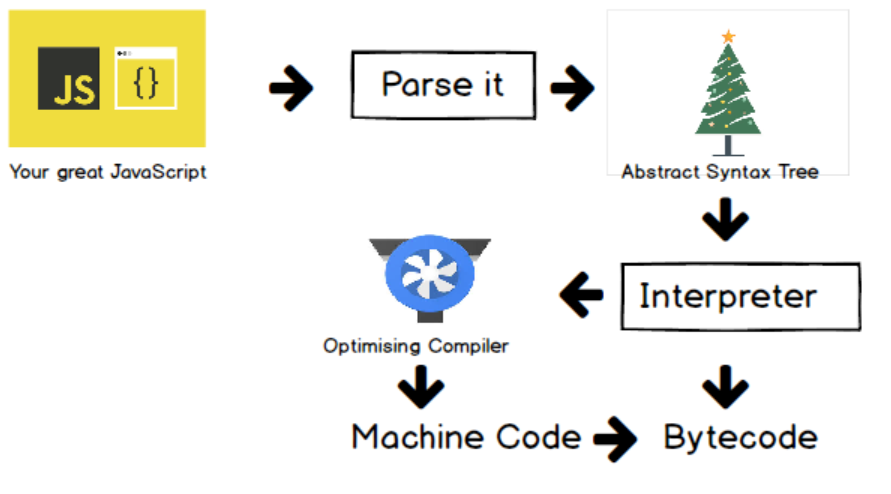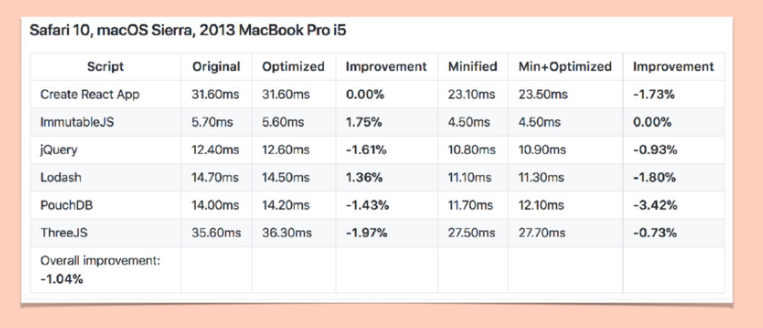1571018162

# The V8 Engine and JavaScript Optimization Tips

## The JavaScript Journey

So what exactly happens when we send our JavaScript to be parsed by the V8 engine (this is after it is minified, uglified and whatever other crazy stuff you do to your JavaScript code)?

I’ve created the following diagram that shows all the steps, we will then discuss each step in detail:In this article we’ll discuss how the JavaScript code gets parsed and how to get as much of your JavaScript to the Optimizing Compiler as possible. The Optimizing Compiler (aka Turbofan) takes our JavaScript code and converts it to high performance Machine Code, so the more code we can give it the faster our application will be. As a side note, the interpreter in Chrome is called Ignition.

## Parsing JavaScript

So the first treatment of our JavaScript code is to parse it. Let’s discuss exactly what parsing is.

There are two phases to parsing which are:

• Eager (full-parse) - this parses each line right away
• Lazy (pre-parse)- do the bare minimum, parse what we need and leave the rest until later

Which is better? It all depends.

Let’s look at some code.

``````// eager parse declarations right away
const a = 1;
const b = 2;

// lazily parse this as we don't need it right away
return a + b;
}

// oh looks like we do need add so lets go back and parse it

``````

So here our variable declarations will be `eager parsed` but then our function is `lazily parsed`. This is great until we get to `add(a, b)` as we need our `add` function right away so it would have been quicker to `eager parse` `add` right away.

To `eager parse` the `add` function right away, we can do:

``````// eager parse declarations right away
const a = 1;
const b = 2;

// eager parse this too
var add = (function(a, b) {
return a + b;
})();

// we can use this right away as we have eager parsed

``````

This is how most modules you use are created. So is eager parsing the best way to go for a performant JavaScript application?

Let’s look at the library optimize-js, which goes through libraries and eager parses all the code. If we look at a popular library like `lodash`, these optimizations are great:

• Without optimize-js: 11.86ms
• With optimize-js: 11.24ms

But it has to be accounted that this is in a Chrome browser environment, on other environments this can shoot us in the foot:So when making these optimizations to your web app, it’s important to test in all the environments your app will be running in.

Another parsing tip is not to nest functions in other functions:

``````// bad way
function sumOfSquares(a, b) {
// this is lazily parsed over and over
function square(num) {
return num * num;
}

return square(a) + square(b);
}

``````

The better way to do this is:

``````function square(num) {
return num * num;
}

// good way
function sumOfSquares(a, b) {
return square(a) + square(b);
}

sumOfSquares(a, b);

``````

In the above case, `square` is only `lazily parsed` once.

## Function Inlining

Chrome will sometimes essentially rewrite your JavaScript, one example of this is inlining a function that is being used.

Let’s take the following code as an example:

``````const square = (x) => { return x * x }

const callFunction100Times = (func) => {
for(let i = 100; i < 100; i++) {
// the func param will be called 100 times
func(2)
}
}

callFunction100Times(square)

``````

The above code will be optimized by the V8 engine as follows:

``````const square = (x) => { return x * x }

const callFunction100Times = (func) => {
for(let i = 100; i < 100; i++) {
// the function is inlined so we don't have
// to keep calling func
return x * x
}
}

callFunction100Times(square)

``````

As you can see from the above, V8 is essentially removing the step where we call `func` and instead inlining the body of `square`. This is very useful as it will improve the performance of our code.

### Function inlining gotcha

There is a little gotcha with this approach, let’s take the following code example:

``````const square = (x) => { return x * x }
const cube = (x) => { return x * x * x }

const callFunction100Times = (func) => {
for(let i = 100; i < 100; i++) {
// the function is inlined so we don't have
// to keep calling func
func(2)
}
}

callFunction100Times(square)
callFunction100Times(cube)

``````

So this time after we have called the `square` function `100` times, we will then call the `cube` function `100` times. Before `cube` can be called, we must first de-optimize the `callFunction100Times` as we have inlined the `square` function body. In cases like this, the `square` function will seem like it’s faster than the `cube` function but what is happening is the de-optimization step makes the execution longer.

## Objects

When it comes to objects, V8 under the hood has a type system to differentiate your objects:

### Monomorphism

The objects have the same keys with no differences.

``````// mono example
const person = { name: 'John' }
const person2 = { name: 'Paul' }

``````

### Polymorphism

The objects share a similar structure with some small differences.

``````// poly example
const person = { name: 'John' }
const person2 = { name: 'Paul', age: 27 }

``````

### Megamorphism

The objects are entirely different and cannot be compared.

``````// mega example
const person = { name: 'John' }
const building = { rooms: ['cafe', 'meeting room A', 'meeting room B'], doors: 27 }

``````

So now we know the different objects in V8, let’s see how V8 optimizes our objects.

### Hidden classes

Hidden classes are how V8 identifies our objects.

Let’s break this down into steps.

We declare an object:

``````const obj = { name: 'John'}

``````

V8 will then declare a `classId` for this object.

``````const objClassId = ['name', 1]

``````

Then our object is created as follows:

``````const obj = {...objClassId, 'John'}

``````

Then when we access the `name` property on our object like so:

``````obj.name

``````

V8 does the following lookup:

``````obj[getProp(obj, name)]

``````

This is process V8 goes through when creating our objects, now let’s see how we can optimize our objects and reuse `classIds`.

## Tips for Creating Objects

If you can, you should declare your properties in the constructor. This will ensure the object structure stays the same so V8 can then optimize your objects.

``````class Point {
constructor(x,y) {
this.x = x
this.y = y
}
}

const p1 = new Point(11, 22) // hidden classId created
const p2 = new Point(33, 44)

``````

You should keep the property order constant, take the following example:

``````const obj = { a: 1 } // hidden class created
obj.b = 3

const obj2 = { b: 3 } // another hidden class created
obj2.a = 1

// this would be better
const obj = { a: 1 } // hidden class created
obj.b = 3

const obj2 = { a: 1 } // hidden class is reused
obj2.b = 3

``````

## General Optimization Tips

So now let’s get into some general tips that will help your JavaScript code be better optimized.

### Fix function argument types

When arguments are being passed to a function it’s important they are the same type. Turbofan will give up trying to optimize your JavaScript after 4 tries if the argument types are different.

Take the following example:

``````function add(x,y) {
return x + y
}

add([],[]) // megamorphic - at this stage, 4+ tries, no optimization will happen

``````

Another tip is to make sure to declare classes in the global scope:

``````// don't do this
function createPoint(x, y) {
class Point {
constructor(x,y) {
this.x = x
this.y = y
}
}

// new point object created every time
return new Point(x,y)
}

function length(point) {
//...
}

``````

## Conclusion

So I hope you learned a few things about how V8 works under the hood and how to write better optimized JavaScript code.

#javascript

## Buddha Community1619518440

## top 30 Python Tips and Tricks for Beginners

Welcome to my Blog , In this article, you are going to learn the top 10 python tips and tricks.

### 8) Check The Memory Usage Of An Object.

#python #python hacks tricks #python learning tips #python programming tricks #python tips #python tips and tricks #python tips and tricks advanced #python tips and tricks for beginners #python tips tricks and techniques #python tutorial #tips and tricks in python #tips to learn python #top 30 python tips and tricks for beginners

1589255577

## The essential JavaScript concepts that you should understand

As a JavaScript developer of any level, you need to understand its foundational concepts and some of the new ideas that help us developing code. In this article, we are going to review 16 basic concepts. So without further ado, let’s get to it.

#javascript-interview #javascript-development #javascript-fundamental #javascript #javascript-tips1610158495

## 7 Simple JavaScript Tips for Optimizing Your Code

I know you care about optimizing your code but sometimes you don’t know how to do it.

Imagine every piece of your code is well optimized, the performance would be great and you can easily investigate your code if having any issue.

So, without any further ado, let’s dive right into the 7 simple JavaScript tips below.

#programming-tips #javascript #programming #javascript-tips

1622207074

## What is JavaScript - Stackfindover - Blog

Who invented JavaScript, how it works, as we have given information about Programming language in our previous article ( What is PHP ), but today we will talk about what is JavaScript, why JavaScript is used The Answers to all such questions and much other information about JavaScript, you are going to get here today. Hope this information will work for you.

## Who invented JavaScript?

JavaScript language was invented by Brendan Eich in 1995. JavaScript is inspired by Java Programming Language. The first name of JavaScript was Mocha which was named by Marc Andreessen, Marc Andreessen is the founder of Netscape and in the same year Mocha was renamed LiveScript, and later in December 1995, it was renamed JavaScript which is still in trend.

## What is JavaScript?

JavaScript is a client-side scripting language used with HTML (Hypertext Markup Language). JavaScript is an Interpreted / Oriented language called JS in programming language JavaScript code can be run on any normal web browser. To run the code of JavaScript, we have to enable JavaScript of Web Browser. But some web browsers already have JavaScript enabled.

Today almost all websites are using it as web technology, mind is that there is maximum scope in JavaScript in the coming time, so if you want to become a programmer, then you can be very beneficial to learn JavaScript.

## JavaScript Hello World Program

In JavaScript, ‘document.write‘ is used to represent a string on a browser.

``````<script type="text/javascript">
document.write("Hello World!");
</script>
``````

## How to comment JavaScript code?

• For single line comment in JavaScript we have to use // (double slashes)
• For multiple line comments we have to use / * – – * /
``````<script type="text/javascript">

//single line comment

/* document.write("Hello"); */

</script>
``````

#javascript #javascript code #javascript hello world #what is javascript #who invented javascript1616670795

## Hire Dedicated JavaScript Developers -Hire JavaScript Developers

It is said that a digital resource a business has must be interactive in nature, so the website or the business app should be interactive. How do you make the app interactive? With the use of JavaScript.